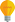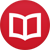# Render LaTeX in Wikipedia using Mathjax

by Jakub MarianTip: See my list of theMost Common Mistakes in English. It will teach you how to avoid mis­takes with com­mas, pre­pos­i­tions, ir­reg­u­lar verbs, and much more.

Let’s face itmathematical expressions rendered as images look ugly, especially in inline formulas. Also, if you, like me, have all Wikipedia articles zoomed in (the text is just way too tiny for a high-resolution screen), the images become even uglier.

Fortunately, there’s now a simple way to fix that. You can render all LaTeX formulas using MathJax, a brilliant javascript tool which renders mathematical expressions directly in browser as text, e.g. $÷{d}{dt}∫_0^t f(x)\,dx = f(t)$. To use MathJax on Wikipedia, do the following:

There used to be an easy way to use MathJax simply by selecting the “MathJax” option in the appearance tab, but the developers of Wikipedia removed this option for some reason. However, it is still easy to use it.

If you use Chrome, you can install an extension that will render all formulas on Wikipedia with MathJax. The advantage of this solution is that it will worke even when you are not logged in.

## Use to following JavaScript code

I am not aware of any such extension for Firefox, but there is a way to make force MathJax rendering for logged users using any browser. To do that, log in and open the following URL (replace YOURUSERNAME with your actual username)

or open the appearance tab in preferences and click on Custom JavaScript under Shared CSS/JavaScript for all wikis.

Now click on Edit in the top menu, copy and paste the following code (developed by Peter Krautzberger) into the editor, and hit Save page:

var mathTags = $('.mwe-math-mathml-a11y'); if (mathTags.length > 0){ window.MathJax = { AuthorInit: function () { MathJax.Hub.Register.StartupHook("End",function () { MathJax.Hub.Queue( function(){ mathTags.removeClass('mwe-math-mathml-a11y');$('.mwe-math-fallback-image-inline').addClass('mwe-math-mathml-a11y');
}
);
});
}
};